# 1386 What You Need to Know About the Multiplication Game

I recently wrote about Hooda Math’s Multiplication Game. There’s a couple of things I didn’t tell you in that post.

First of all, you don’t have to use a computer to play the game. (However, using one the first time you play will help you understand how to play). You can print a game board to play. I’ve created a game board below that you could use. Each player can use different items such as beads, pennies, nickels, and dimes as markers to mark the factors used and to claim the resulting products on the game board.The second thing you should know is that getting four squares in a row, horizontally, vertically or diagonally is NOT equally likely every place on the board. If one particular number is all you need to get a win, you are less likely to get that number if it only has one factor (like the numbers marked in yellow have). As far as this game is concerned, the products have the number of factors that I’ve indicated, even though in reality most of them have more than that.

You can’t win unless your opponent gives you one of the factors you need to claim that winning space. If 4 of the 9 possible factors will get it for you, the odds are much better your opponent will give you what you need than if only 1 of the 9 possible factors will do it.

If you know which numbers have four possible factors, you may have an advantage over someone who thinks this game is really just a variation of tic-tac-toe. Of course, those products with four factors could also make you more likely to get blocked as well! And if you use my colorful game board, your opponent will know just as much as you do about how many ways they can get each square.

### 1386 Factor Cake:

Since the biggest prime factor of 1386 is 11, it makes an especially festive factor cake!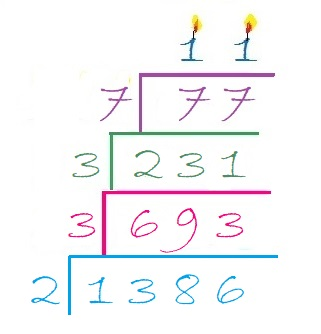### Factors of 1386:

Now I’ll share some information about the number 1386:

• 1386 is a composite number.
• Prime factorization: 1386 = 2 × 3 × 3 × 7 × 11, which can be written 1386 = 2 × 3² × 7 × 11
• 1386 has at least one exponent greater than 1 in its prime factorization so √1386 can be simplified. Taking the factor pair from the factor pair table below with the largest square number factor, we get √1386 = (√9)(√154) = 3√154
• The exponents in the prime factorization are 1, 2, 1, and 1. Adding one to each exponent and multiplying we get (1 + 1)(2 + 1)(1 + 1)(1 + 1) = 2 × 3 × 2 × 2 = 24. Therefore 1386 has exactly 24 factors.
• The factors of 1386 are outlined with their factor pair partners in the graphic below.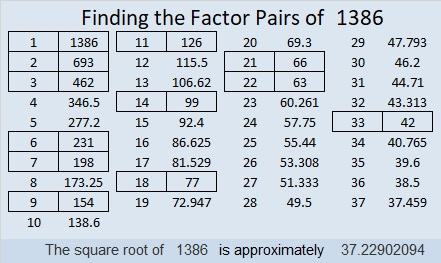### Sum-Difference Puzzle:

1386 has twelve factor pairs. One of the factor pairs adds up to 85, and a different one subtracts to 85. If you can identify those factor pairs, then you can solve this puzzle!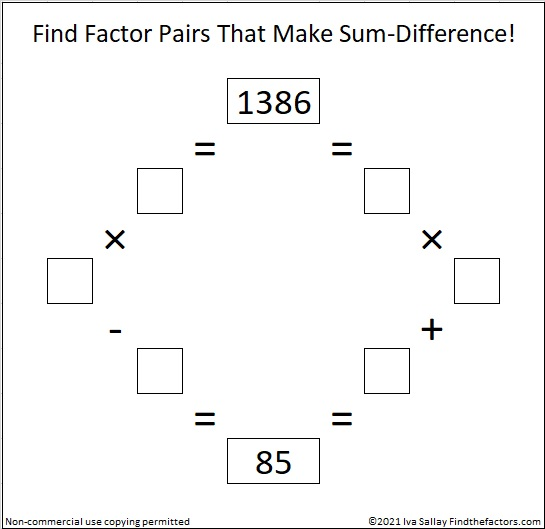### One More Fact about the Number 1386:

OEIS.org also noted that 1 + 3⁴ + 8 + 6⁴ = 1386.

# 1350 Logic is at the Heart of This Puzzle

### Today’s Puzzle:

By simply changing two clues of that recently published puzzle that I rejected, I was able to create a love-ly puzzle that can be solved entirely by logic. Can you figure out where to put the numbers from 1 to 12 in each of the four outlined areas that divide the puzzle into four equal sections? If you can, my heart might just skip a beat!

If you need some tips on how to get started on this puzzle, check out this video:

### Factors of 1350:

• 1350 is a composite number.
• Prime factorization: 1350 = 2 × 3 × 3 × 3 × 5 × 5, which can be written 1350 = 2 × 3³ × 5²
• The exponents in the prime factorization are 1, 3 and 2. Adding one to each and multiplying we get (1 + 1)(3 + 1)(2 + 1) = 2 × 4 × 3 = 24. Therefore 1350 has exactly 24 factors.
• Factors of 1350: 1, 2, 3, 5, 6, 9, 10, 15, 18, 25, 27, 30, 45, 50, 54, 75, 90, 135, 150, 225, 270, 450, 675, 1350
• Factor pairs: 1350 = 1 × 1350, 2 × 675, 3 × 450, 5 × 270, 6 × 225, 9 × 150, 10 × 135, 15 × 90, 18 × 75, 25 × 54, 27 × 50 or 30 × 45
• Taking the factor pair with the largest square number factor, we get √1350 = (√225)(√6) = 15√6 ≈ 36.74235

### Sum-Difference Puzzles:

6 has two factor pairs. One of those pairs adds up to 5, and the other one subtracts to 5. Put the factors in the appropriate boxes in the first puzzle.

1350 has twelve factor pairs. One of the factor pairs adds up to ­75, and a different one subtracts to 75. If you can identify those factor pairs, then you can solve the second puzzle!The second puzzle is really just the first puzzle in disguise. Why would I say that?

### More about the Number 1350:

1350 is the sum of consecutive prime numbers two ways:
It is the sum of the fourteen prime numbers from 67 to 131, and
673 + 677 = 1350

1350 is the hypotenuse of two Pythagorean triples:
810-1080-1350 which is (3-4-5) times 270
378-1296-1350 which is (7-24-25) times 54

1350 is also the 20th nonagonal number because 20(7 · 20 – 5)/2 = 1350

# How Far Away Is 1344 from the Nearest Prime Number?

### The Distance 1344 is from the Nearest Prime Number:

1344 is 17 numbers away from the nearest prime number. 17-away is a new record for distance to the closest prime number!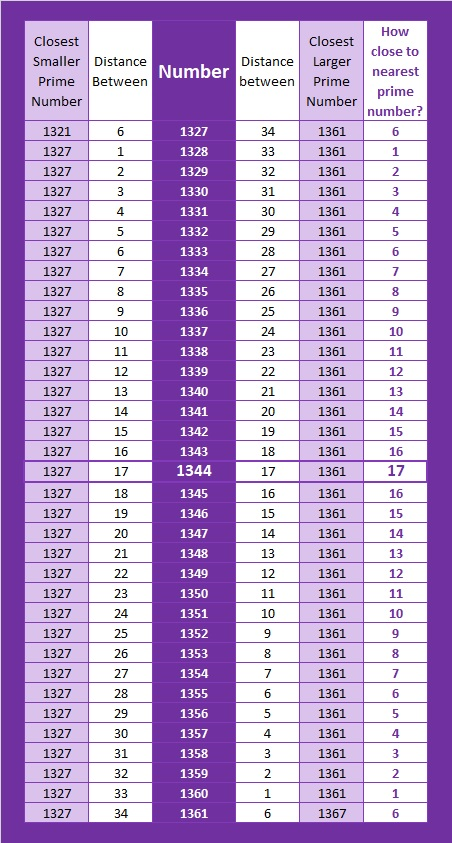1344 will hold that record until prime number 2179 claims it with being 18-away from the nearest prime:
2161–(18 composite numbers)–2179–(24 composite numbers)–2203

OEIS.org mentioned the previous two numbers, 1342 and 1343, breaking the 15-away and the 16 away records respectively, but didn’t mention this one. I guess it considered 1344 being the order of a perfect group to be more important.

### Factors of 1344:

• 1344 is a composite number.
• Prime factorization: 1344 = 2 × 2 × 2 × 2 × 2 × 2 × 3 × 7, which can be written 1344 = 2⁶ × 3 × 7
• 1344 has at least one exponent greater than 1 in its prime factorization so √1344 can be simplified. Taking the factor pair from the factor pair table below with the largest square number factor, we get √1344 = (√64)(√21) = 8√21
• The exponents in the prime factorization are 6, 1 and 1. Adding one to each exponent and multiplying we get (6 + 1)(1 + 1)(1 + 1) = 7 × 2 × 2 = 28. Therefore 1344 has exactly 28 factors.
• The factors of 1344 are outlined with their factor pairs in the graphic below.### Sum-Difference Puzzles:

84 has six factor pairs. One of those pairs adds up to 25, and another one subtracts to 25. Put the factors in the appropriate boxes in the first puzzle.

1344 has fourteen factor pairs. One of the factor pairs adds up to ­100, and a different one subtracts to 100. If you can identify those factor pairs, then you can solve the second puzzle!The second puzzle is really just the first puzzle in disguise. Why would I say that?

# How I Knew Immediately that a Factor Pair of 1224 is . . .

### Check Out This Pattern!

12 = 3 × 4 and 24 is one less than 25. Those two facts helped me to know right away that 35² = 1225 and 34 × 36 = 1224. Study the patterns in the chart below and you will likely be able to remember all of the multiplication facts listed in it!a² – b² = (a – b)(a + b)
You may remember how to factor that from algebra class. Here when b = 1, it has a practical application that can allow you to amaze your friends and family with your mental calculating abilities!

I’ve only typed a small part of that infinite pattern chart. For example, if you know that 19 × 20 = 380, then you can also know that 195² = 38025 and 194 × 196 = 38024.

Also because of that chart, I know that 3.5² = 12.25 and 3.4 × 3.6 = 12.24
(Also (3½)² = 12¼, but 2½  × 4½ = 11¼ because 3-1 = 2, 3+1 = 4, 12-1 = 11
thus 2.5 × 4.5 = 11.25 and 2½  × 4½ = 11¼)

You could also let b = 2 so b² = 4. Then 25 – 4 = 21, and you could know facts like
33 × 37 = 1221 or 193 ×  197 = 38021.

I hope you have a wonderful time being a calculating genius!

### A Factor Tree for 1224:

When a number has many factors, I often will make a forest of factor trees for that number, but today I just want us to enjoy this one tree for 34 × 36 = 1224.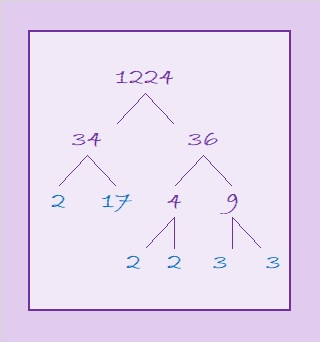### Factors of 1224:

Now I’ll share some other facts about the number 1224:

• 1224 is a composite number.
• Prime factorization: 1224 = 2 × 2 × 2 × 3 × 3 × 17, which can be written 1224 = 2³ × 3² × 17
• The exponents in the prime factorization are 2, 3 and 1. Adding one to each and multiplying we get (3 + 1)(2 + 1)(1 + 1) = 4 × 3 × 2 = 24. Therefore 1224 has exactly 24 factors.
• Factors of 1224: 1, 2, 3, 4, 6, 8, 9, 12, 17, 18, 24, 34, 36, 51, 68, 72, 102, 136, 153, 204, 306, 408, 612, 1224
• Factor pairs: 1224 = 1 × 1224, 2 × 612, 3 × 408, 4 × 306, 6 × 204, 8 × 153, 9 × 136, 12 × 102, 17 × 72, 18 × 68, 24 × 51 or 34 × 36
• Taking the factor pair with the largest square number factor, we get √1224 = (√36)(√34) = 6√34 ≈ 34.98571### Sum-Difference Puzzle:

1224 has twelve factor pairs. One of the factor pairs adds up to 145, and a different one subtracts to 145. If you can identify those factor pairs, then you can solve this puzzle!If finding a sum and a difference equalling 3-digit 145 is too challenging, the chart below will be helpful.### More about the Number 1224:

1224 is also the sum of two squares:
30² + 18² = 1224

1224 is the hypotenuse of a Pythagorean triple:
576-1080-1224 which is (8-15-17) times 72
That triple can also be calculated from 30² – 18², 2(30)(18), 30² + 18²

293 + 307 + 311 + 313 = 1224 making 1224 the sum of four consecutive prime numbers.

# How to Simplify √1176

### Simplifying √1176:

About 83% of numbers whose square root can be simplified are divisible by 4 or by 9 or by both. It’s easy to see rather quickly if a number is divisible by either of them. I want to find square roots by doing as few divisions as possible. It is easier to divide a number by 4 than it is to divide it by 2 twice. Likewise, it is easier to divide a number by 9 than it is to divide it by 3 twice.

1176 is divisible by 4 because the number formed from its last two digits, 76, is divisible by 4. So let’s do that division:
1176÷4=294.

Can we divide 294 by 4? We can quickly see that 94 is not divisible by 4, so 294 is not divisible by 4. Let’s check to see if it is divisible by nine: 2 + 9 + 4 = 15, which is not divisible by 9 but is by 3. That means that 294 is not divisible by 9, but it is divisible by 3.

Since 294 is an even number that is divisible by 3, I will save time and make fewer mistakes if I just divide it by 6 instead of by 2 and then by 3.
294÷6 = 49

49 is a perfect square so I know I’ve done enough dividing for this particular number. I’ve made a gif to illustrate the steps I’ve just explained:make science GIFs like this at MakeaGif

### Factor Trees for 1176:

By doing just a little bit of arithmetic in my head, I can also tell by looking at that cake that the prime factorization of 1176 is 2³ × 3 × 7².

Some people still prefer to use factor trees to find square roots. Here are a few of its MANY possible trees.### Factors of 1176:

• 1176 is a composite number.
• Prime factorization: 1176 = 2 × 2 × 2 × 3 × 7 × 7, which can be written 1176 = 2³ × 3 × 7²
• The exponents in the prime factorization are 3, 1 and 2. Adding one to each and multiplying we get (3 + 1)(1 + 1)(2 + 1) = 4 × 2 × 3 = 24. Therefore 1176 has exactly 24 factors.
• Factors of 1176: 1, 2, 3, 4, 6, 7, 8, 12, 14, 21, 24, 28, 42, 49, 56, 84, 98, 147, 168, 196, 294, 392, 588, 1176
• Factor pairs: 1176 = 1 × 1176, 2 × 588, 3 × 392, 4 × 294, 6 × 196, 7 × 168, 8 × 147, 12 × 98, 14 × 84, 21 × 56, 24 × 49 or 28 × 42
• Taking the factor pair with the largest square number factor, we get √1176 = (√196)(√6) = 14√6 ≈ 34.29286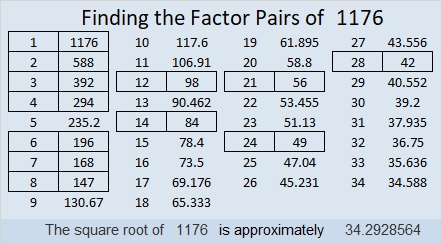### Sum-Difference Puzzles:

6 has two factor pairs. One of those pairs adds up to 5, and the other one subtracts to 5. Put the factors in the appropriate boxes in the first puzzle.

1176 has twelve factor pairs. One of the factor pairs adds up to ­70, and a different one subtracts to 70. If you can identify those factor pairs, then you can solve the second puzzle!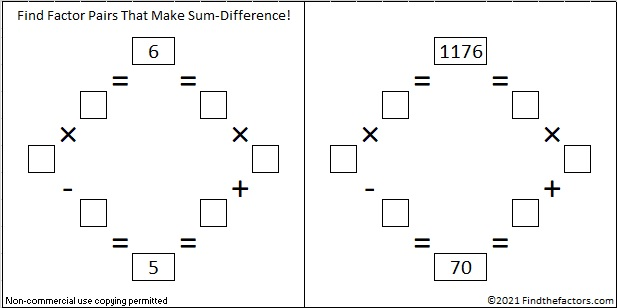The second puzzle is really just the first puzzle in disguise. Why would I say that?

### More about the Number 1176:

1176 is the sum of the first 48 numbers so we say it the 48th triangular number. We know it is the sum of the first 48 numbers because (48 × 49)/2 = 1176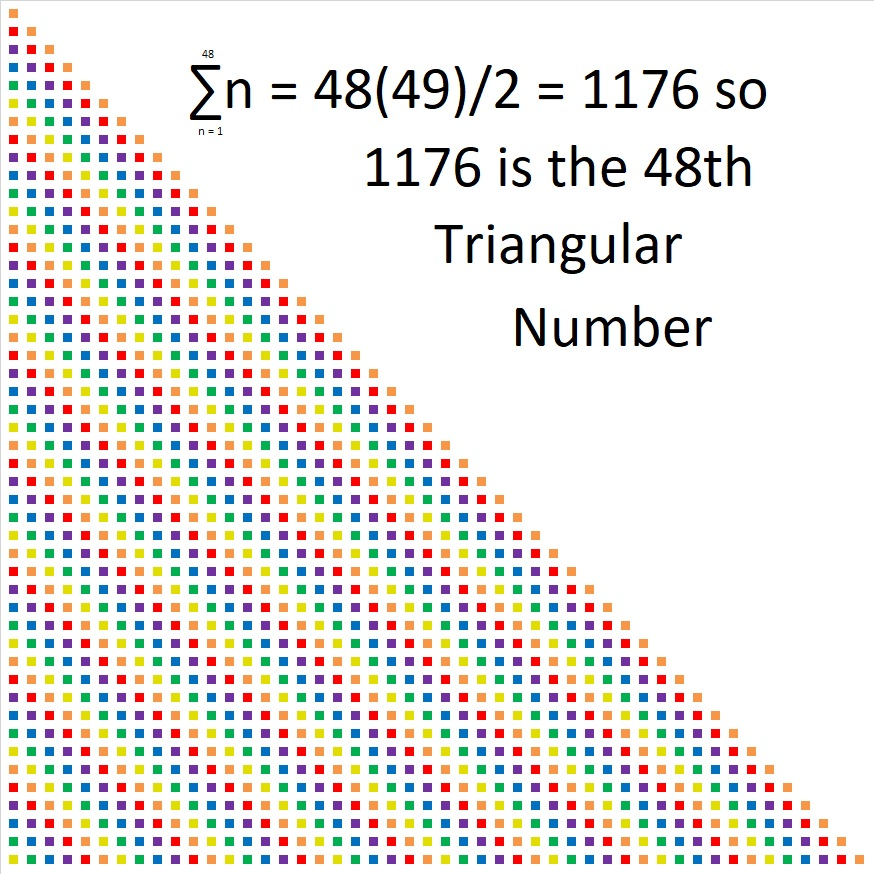1176 looks interesting in a few other bases:
It’s 3300 in BASE 7 because 3(7³ + 7²) = 3(392) = 1176,
6C6 in BASE 13 (C is 12 base 10) because 6(13²) + 12(13) + 6(1) = 1176,
600 in BASE 14 because 6(14²) = 1176,
1M1 in BASE 25 (M is 22 base 10) because 25² + 22(25) + 1 = 1176
and it looks like one of its factors, 196, in BASE 30
because 1(30²) + 9(30) + 6(1) = 1176

# STOP! Look How Cool a Number 1080 Is!

### STOP Sign Geometry Fact:

What can I tell you about the number 1080?  Lots of things!

The sum of the interior angles of an eight-sided polygon such as a stop sign is 1080°.The sum of the interior angles of an octagon is 6(180°) = 1080°. Convex or Concave, it doesn’t matter, the sum of those interior angles of an eight-sided polygon will still be 1080°, as illustrated below:### Factor Trees for 1080:

Here are a couple of the MANY possible factor trees for 1080: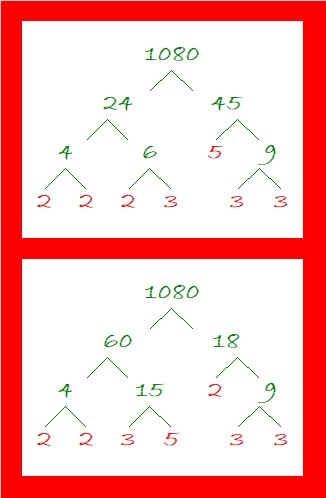### Factors of 1080:

There is only one number less than 1080 that has as many factors as 1080 does. What was that number? 840. How many factors does1080 have? 32. Wow!

• 1080 is a composite number.
• Prime factorization: 1080 = 2 × 2 × 2 × 3 × 3 × 3 × 5, which can be written 1080 = 2³ × 3³ × 5.
• The exponents in the prime factorization are 3, 3, and 1. Adding one to each and multiplying we get (3 + 1)(3 + 1)(1 + 1) ) = 4 × 4 × 2 = 32. Therefore 1080 has exactly 32 factors.
• Factors of 1080: 1, 2, 3, 4, 5, 6, 8, 9, 10, 12, 15, 18, 20, 24, 27, 30, 36, 40, 45, 54, 60, 72, 90, 108, 120, 135, 180, 216, 270, 360, 540, 1080
• Factor pairs: 1080 = 1 × 1080, 2 × 540, 3 × 360, 4 × 270, 5 × 216, 6 × 180, 8 × 135, 9 × 120, 10 × 108, 12 × 90, 15 × 72, 18 × 60, 20 × 54, 24 × 45, 27 × 40 or 30 × 36
• Taking the factor pair with the largest square number factor, we get √1080 = (√36)(√30) = 6√30 ≈ 32.863351080 has 28 composite factors and is the smallest number that can make that claim. (Of its 32 factors, all are composite numbers except 1, 2, 3, and 5). That’s more than 840’s 27 composite factors. (Its 32 factors minus 1, 2, 3, 5, and 7)

### Sum Difference Puzzles:

30 has four factor pairs. One of those pairs adds up to 13, and  another one subtracts to 13. Put the factors in the appropriate boxes in the first puzzle.

1080 has sixteen factor pairs. One of the factor pairs adds up to ­78, and a different one subtracts to 78. If you can identify those factor pairs, then you can solve the second puzzle!The second puzzle is really just the first puzzle in disguise. Why would I say that?

### Other Facts about the Number 1080:

1080 is the sum of these four consecutive prime numbers:
263 + 269 + 271 + 277 = 1080

1080 is the sum of four consecutive powers of three:
3⁶ + 3⁵ + 3⁴ + 3³ = 1080

1080 is the hypotenuse of a Pythagorean triple:
648-864-1080 which is (3-4-5) times 216

Note that 5(6³) = 5(216) = 1080 so 1080 is 500 in BASE 6.
It’s palindrome 252 in BASE 22 because 2(22²) + 5(22) + 2(1) = 1080,
UU in BASE 35 (U is 30 base 10) because 30(35) + 30(1) = 30(36) = 1080,
and it’s U0 in BASE 36 because 30(36) = 1080

And now I’ll STOP writing about how cool 1080 is.

# Level 2 and Simplifying √1014

### Today’s Puzzle:

Have you memorized a basic multiplication table? If you have, then you can solve this puzzle. The numbers being multiplied together aren’t where they are in a regular multiplication table, but you can still easily figure out where they need to go. There is only one solution. I bet you can find it!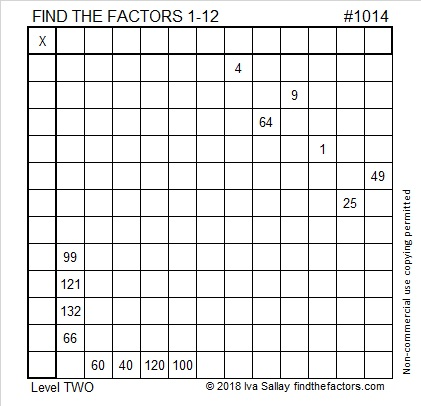Print the puzzles or type the solution in this excel file: 12 factors 1012-1018

### Simplifying √1014:

If I wanted to find √1014, I would first check to see if it were divisible by 4 or by 9 because most numbers whose square roots can be simplified are divisible by 4 or by 9 or both.
1014 isn’t divisible by 4 because 14 isn’t divisible by 4.
It isn’t divisible by 9 because 1 + 0 + 1 + 4 = 6, and 6 is not divisible by 9.
However, it is divisible by both 2 and 3 and thus also by 6. Since most people are less likely to make a mistake dividing by 6 in ONE step instead of two, I would make a little division cake and do that division first:Recognizing that 169 is a perfect square, I would then take the square root of everything on the outside of my little cake. (√6)(√169) = 13√6

### Factors of 1014:

• 1014 is a composite number.
• Prime factorization: 1014 = 2 × 3 × 13 × 13, which can be written 1014 = 2 × 3 × 13²
• The exponents in the prime factorization are 1, 1, and 2. Adding one to each and multiplying we get (1 + 1)(1 + 1)(2 + 1) = 2 × 2 × 3 = 12. Therefore 1014 has exactly 12 factors.
• Factors of 1014: 1, 2, 3, 6, 13, 26, 39, 78, 169, 338, 507, 1014
• Factor pairs: 1014 = 1 × 1014, 2 × 507, 3 × 338, 6 × 169, 13 × 78, or 26 × 39,
• Taking the factor pair with the largest square number factor, we get √1014 = (√169)(√6) = 13√6 ≈ 31.84337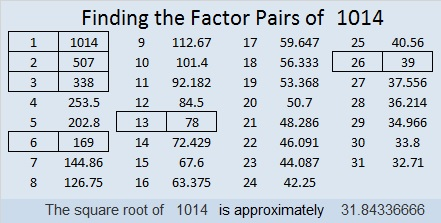### Sum-Difference Puzzles:

6 has two factor pairs. One of those pairs adds up to 5, and the other one subtracts to 5. Put the factors in the appropriate boxes in the first puzzle.

1014 has six factor pairs. One of the factor pairs adds up to ­65, and a different one subtracts to 65. If you can identify those factor pairs, then you can solve the second puzzle!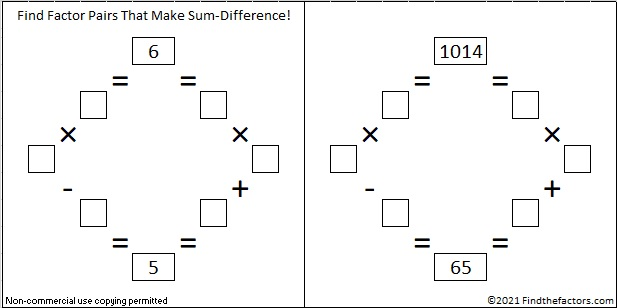The second puzzle is really just the first puzzle in disguise. Why would I say that?

### More about the Number 1014:

Because 13² is one of its factors, 1014 is the hypotenuse of two Pythagorean triples:
714-720-1014 which is 6 times (119-120-169),
390-936-1014 which is (5-12-13) times 78.

1014 looks interesting when written in some other bases:
It’s 600 in BASE 13 because 6(13²) = 6 (169) = 1014,
and 222 in BASE 22 because 2(22²) + 2(22) + 2(1) = 2(484 + 22 + 1) = 2(507) = 1014.

# Applying Divisibility Rules to 924

### Divisibility Rules and 924:

Let’s apply some basic divisibility rules to find some of the factors of 924:

1. Like every other counting number, 924 is divisible by 1.
2. Since 924 is even, it is divisible by 2.
3. 9 + 2 + 4 = 15, a number divisible by 3, so 924 is divisible by 3.
4. Its last two digits, 24, is divisible by 4, so 924 is divisible by 4.
5. Its last digit isn’t 0 or 5, so 924 is NOT divisible by 5.
6. 924 is even and divisible by 3, so it is also divisible by 6.
7. Since 92-2(4) = 84, a number divisible by 7, we know that 924 is also divisible by 7.
8. Since its last two digits are divisible by 8, and the third to the last digit, 9, is odd, 924 is NOT divisible 8.
9. 9 + 2 + 4 = 15, a number not divisible by 9, so 924 is NOT divisible by 9.
10. The last digit is not 0, so 924 is NOT divisible by 10.
11. 9 – 2 + 4 = 11, so 924 is divisible by 11.

Thus, 1, 2, 3, 4, 6, 7, and 11 are all factors of 924.

### Factor Cake for 924:

You can see its prime factors easily on the outside of its festive prime factor cake:### Factors of 924:

• 924 is a composite number.
• Prime factorization: 924 = 2 x 2 x 3 x 7 x 11, which can be written 924 = 2² x 3 x 7 x 11
• The exponents in the prime factorization are 2, 1, 1, and 1. Adding one to each and multiplying we get (2 + 1)(1 + 1)(1 + 1)(1 + 1) = 3 x 2 x 2 x 2 = 24. Therefore 924 has exactly 24 factors.
• Factors of 924: 1, 2, 3, 4, 6, 7, 11, 12, 14, 21, 22, 28, 33, 42, 44, 66, 77, 84, 132, 154, 231, 308, 462, 924
• Factor pairs: 924 = 1 x 924, 2 x 462, 3 x 308, 4 x 231, 6 x 154, 7 x 132, 11 x 84, 12 x 77, 14 x 66, 21 x 44, 22 x 42, or 28 x 33,
• Taking the factor pair with the largest square number factor, we get √924 = (√4)(√231) = 2√231 ≈ 30.3973683.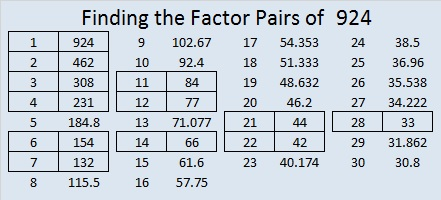### Sum Difference Puzzle:

924 has twelve factor pairs. One of the factor pairs adds up to 65, and a different one subtracts to 65. If you can identify those factor pairs, then you can solve this puzzle!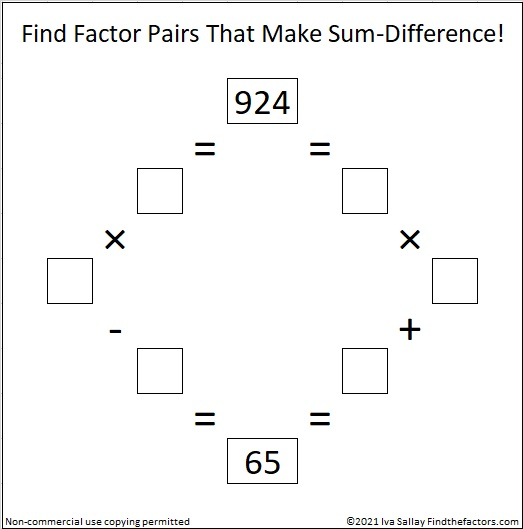### More about the Number 924:

You may have seen one of its many possible factor trees contained in the first frame of this factor tree for 852,852 from my previous post:924 is in the very center of the 12th row of Pascal’s triangle because 12!/(6!6!) = 924.924 is the sum of consecutive prime numbers: 461 + 463 = 924

I like 924 written in some other bases:
770 BASE 11
336 BASE 17
220 BASE 21
SS BASE 32, S is 28
S0 BASE 33

924 has several sets of consecutive factors. Besides being divisible by the 1st, 2nd, and 3rd triangular numbers (1, 3, and 6), those consecutive factors mean the following:

• 924 is divisible by the 6th triangular number, 21, which is 6(7)/2.
• 924 is divisible by the 11th triangular number, 66, which is 11(12)/2.
• 924 is divisible by the 21st triangular number, 231, which is 21(22)/2.

# 864 Factor Trees

### Factor Trees for 864:

The prime factorization of 864 is 2⁵ × 3³. The sum of the exponents is 5 + 3 = 8. Since 8 is a power of 2,  a couple of 864’s factor trees are full and well-balanced:All of those prime factors lined up in numerical order. That didn’t happen for the next one, but it still makes a good looking tree, and all the prime factors are easy to find.Is it possible to make a factor tree for 864 that hardly looks like a tree and isn’t as easy to find all the prime factors? Yes, it is. Here’s an example: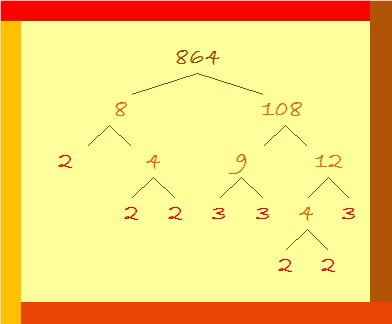### Factors of 864:

• 864 is a composite number.
• Prime factorization: 864 = 2 × 2 × 2 × 2 × 2 × 3 × 3 × 3, which can be written 864 = 2⁵ × 3³
• The exponents in the prime factorization are 5 and 3. Adding one to each and multiplying we get (5 + 1)(3 + 1) = 6 × 4 = 24. Therefore 864 has exactly 24 factors.
• Factors of 864: 1, 2, 3, 4, 6, 8, 9, 12, 16, 18, 24, 27, 32, 36, 48, 54, 72, 96, 108, 144, 216, 288, 432, 864
• Factor pairs: 864 = 1 × 864, 2 × 432, 3 × 288, 4 × 216, 6 × 144, 8 × 108, 9 × 96, 12 × 72, 16 × 54, 18 × 48, 24 × 36, or 27 × 32
• Taking the factor pair with the largest square number factor, we get √864 = (√144)(√6) = 12√6 ≈ 29.3938769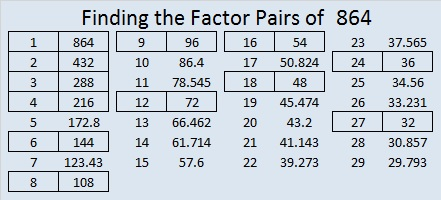### Sum-Difference Puzzles:

6 has two factor pairs. One of those pairs adds up to 5, and the other one subtracts to 5. Put the factors in the appropriate boxes in the first puzzle.

864 has twelve factor pairs. One of the factor pairs adds up to ­60, and a different one subtracts to 60. If you can identify those factor pairs, then you can solve the second puzzle!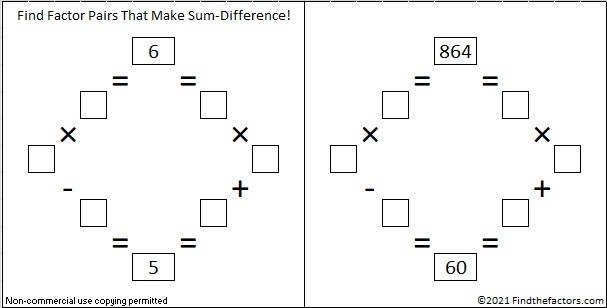### More Facts about the Number 864:

864 looked interesting to me in a few other bases:

• 4000 BASE 6 because 4(6³) = 864
• 600 BASE 12 because 6(12²) = 864
• RR BASE 31 (R is 27 base 10) because 27(31) + 27(1) = 27(32) = 864
• OO BASE 35 (O is 24 base 10) because 24(35) + 24(1) = 24(36) = 864
• O0 BASE 36 (Oh zero) because 24(36) + 0(1) = 864

864 is the sum of the 20 prime numbers from 7 to 83.

131 + 137 + 139 + 149 + 151 + 157 = 864; that’s six consecutive primes.

431 + 433 = 864; that’s the sum of twin primes.

864 is in this cool pattern:# 756 and Level 3

### Today’s Puzzle: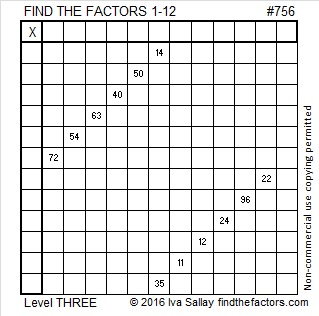Print the puzzles or type the solution on this excel file: 12 Factors 2016-01-25

A Logical Approach to solve a FIND THE FACTORS puzzle: Find the column or row with two clues and find their common factor. (None of the factors are greater than 12.)  Write the corresponding factors in the factor column (1st column) and factor row (top row).  Because this is a level three puzzle, you have now written a factor at the top of the factor column. Continue to work from the top of the factor column to the bottom, finding factors and filling in the factor column and the factor row one cell at a time as you go.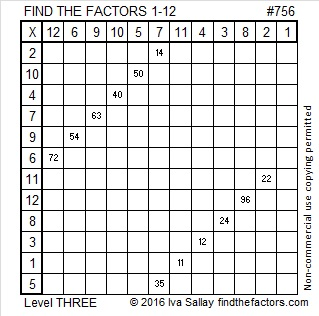### Factor Trees for 756:

756 has many factors and, therefore, it has many possible factor trees. Here are three of them:### Factors of 756:

• 756 is a composite number.
• Prime factorization: 756 = 2 x 2 x 3 x 3 x 3 x 7, which can be written 756 = 2² x 3³ x 7
• The exponents in the prime factorization are 2, 3 and 1. Adding one to each and multiplying we get (2 + 1)(3 + 1)(1 + 1) = 3 x 4 x 2 = 24. Therefore 756 has exactly 24 factors.
• Factors of 756: 1, 2, 3, 4, 6, 7, 9, 12, 14, 18, 21, 27, 28, 36, 42, 54, 63, 84, 108, 126, 189, 252, 378, 756
• Factor pairs: 756 = 1 x 756, 2 x 378, 3 x 252, 4 x 189, 6 x 126, 7 x 108, 9 x 84, 12 x 63, 14 x 54, 18 x 42, 21 x 36 or 27 x 28
• Taking the factor pair with the largest square number factor, we get √756 = (√21)(√36) = 6√21 ≈ 27.495454.### Sum-Difference Puzzles:

84 has six factor pairs. One of those pairs adds up to 25, and another one subtracts to 25. Put the factors in the appropriate boxes in the first puzzle.

756 has twelve factor pairs. One of the factor pairs adds up to ­75, and a different one subtracts to 75. If you can identify those factor pairs, then you can solve the second puzzle!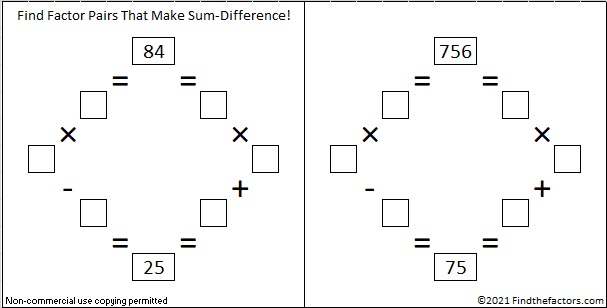The second puzzle is really just the first puzzle in disguise. Why would I say that?

### More about the Number 756:

The last two digits of 756 is divisible by 4 so 756 is divisible by 4.

756 is formed from 3 consecutive numbers (5, 6, 7) so it is divisible by 3. The middle number is divisible by 3 so 756 is also divisible by 9.

756 can be written as the sum of consecutive numbers seven ways:

• 251 + 252 + 253 = 756; that’s 3 consecutive numbers.
• 105 + 106 + 107 + 108 + 109 + 110 + 111 = 756; that’s 7 consecutive numbers.
• 91 + 92 + 93 + 94 + 95 + 96 + 97 + 98 = 756; that’s 8 consecutive numbers.
• 80 + 81 + 82 + 83 + 84 + 85 + 86 + 87 + 88 = 756; that’s 9 consecutive numbers.
• 26 + 27 + 28 + 29 + 30 + 31 + 32 + 33 + 34 + 35 + 36 + 37 + 38 + 39 + 40 + 41 + 42 + 43 + 44 + 45 + 46 = 756; that’s 21 consecutive numbers.
• 20 + 21 + 22 + 23 + 24 + 25 + 26 + 27 + 28 + 29 + 30 + 31 + 32 + 33 + 34 + 35 + 36 + 37 + 38 + 39 + 40 + 41 + 42 + 43 = 756; that’s 24 consecutive numbers.
• 15 + 16 + 17 + 18 + 19 + 20 + 21 + 22 + 23 + 24 + 25 + 26 + 27 + 28 + 29 + 30 + 31 + 32 + 33 + 34 + 35 + 36 + 37 + 38 + 39 + 40 + 41  = 756; that’s 27 consecutive numbers.

756 is also the sum of six consecutive prime numbers: 109 + 113 + 127+ 131 + 137+ 139 = 756.

756 can be written as the sum of three squares four different ways. (Notice that all of the squares are even):

• 26² + 8² + 4² = 756
• 24² + 12² + 6² = 756
• 22² + 16² + 4² = 756
• 20² + 16² + 10² = 756

756 is a palindrome in two other bases:

• 11011 BASE 5; note that 1(625) + 1(125) + 0(25) + 1(5) + 1(1) = 756.
• LL BASE 35 (L is 21 base 10); note that 21(35) + 21(1) = 756.